# 数据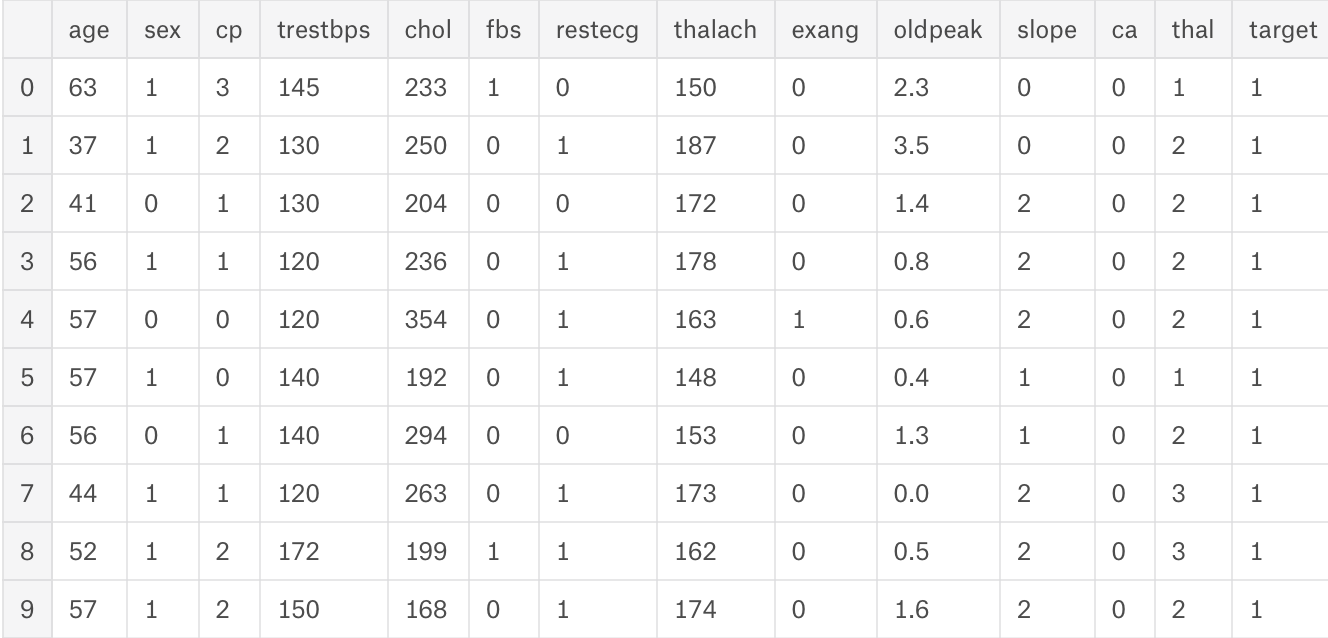# 部分实现细节

## 数据筛选——pandas自带的one-hot实现

dt = pd.get_dummies(dt, drop_first=True)

pandas中自带的one-hot方式实现，drop_first=True是为了减少数据冗余，因为对性别来说一个是1了之后，就可以去定是0的是另一种性别。对与3分类而言同样全0可以表示被删除的另一种情况。

## 可视化决策树方法

X_train, X_test, y_train, y_test = train_test_split(dt.drop('target', 1), dt['target'], test_size = .2, random_state=10) #split the data

model = RandomForestClassifier(max_depth=5)
model.fit(X_train, y_train)


estimator = model.estimators_
feature_names = [i for i in X_train.columns]

y_train_str = y_train.astype('str')
y_train_str[y_train_str == '0'] = 'no disease'
y_train_str[y_train_str == '1'] = 'disease'
y_train_str = y_train_str.values

export_graphviz(estimator, out_file='tree.dot',
feature_names = feature_names,
class_names = y_train_str,
rounded = True, proportion = True,
label='root',
precision = 2, filled = True)

from subprocess import call
call(['dot', '-Tpng', 'tree.dot', '-o', 'tree.png', '-Gdpi=600'])

from IPython.display import Image
Image(filename = 'tree.png')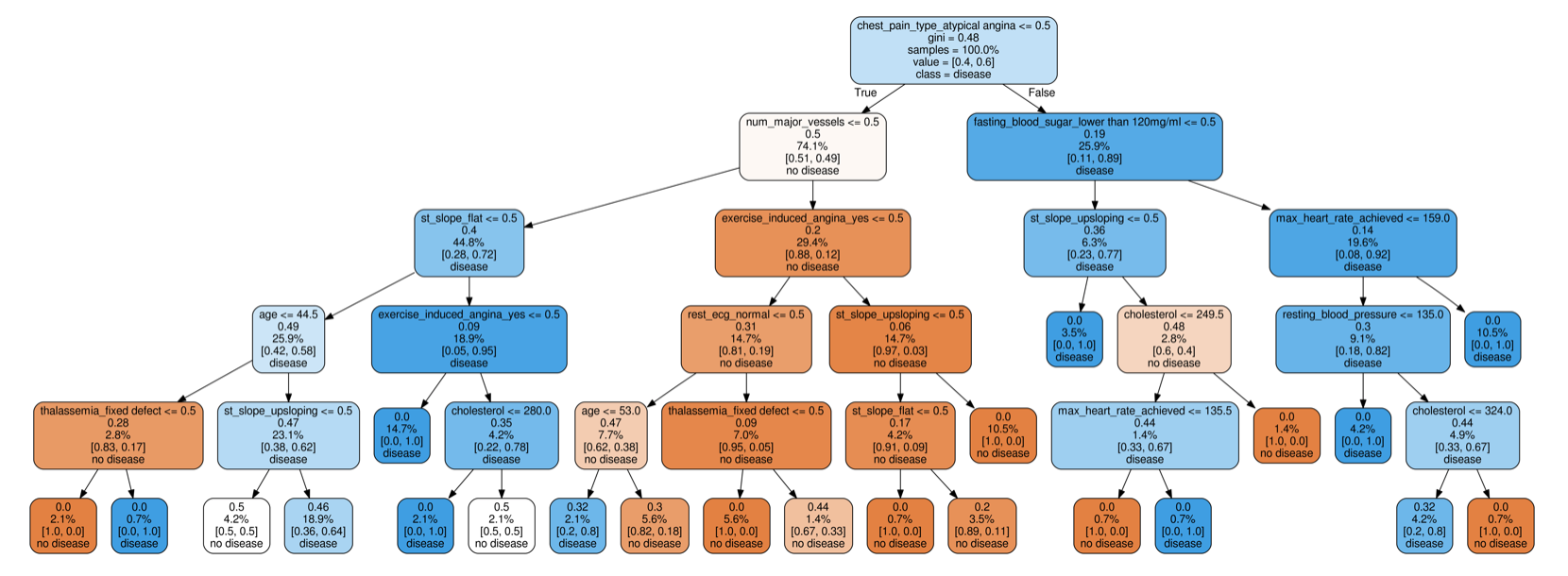from sklearn.ensemble import RandomForestClassifier #for the model
from sklearn.tree import export_graphviz #plot tree


## 混淆矩阵

from sklearn.metrics import roc_curve, auc #for model evaluation
from sklearn.metrics import classification_report #for model evaluation
from sklearn.metrics import confusion_matrix #for model evaluation

confusion_matrix = confusion_matrix(y_test, y_pred_bin)
total=sum(sum(confusion_matrix))

sensitivity = confusion_matrix[0,0]/(confusion_matrix[0,0]+confusion_matrix[1,0])
print('Sensitivity : ', sensitivity )

specificity = confusion_matrix[1,1]/(confusion_matrix[1,1]+confusion_matrix[0,1])
print('Specificity : ', specificity)


array([[28,  7],
[ 3, 23]])

Sensitivity :  0.9032258064516129
Specificity :  0.7666666666666667


ROC曲线：

fpr, tpr, thresholds = roc_curve(y_test, y_pred_quant)

fig, ax = plt.subplots()
ax.plot(fpr, tpr)
ax.plot([0, 1], [0, 1], transform=ax.transAxes, ls="--", c=".3")
plt.xlim([0.0, 1.0])
plt.ylim([0.0, 1.0])
plt.rcParams['font.size'] = 12
plt.title('ROC curve for diabetes classifier')
plt.xlabel('False Positive Rate (1 - Specificity)')
plt.ylabel('True Positive Rate (Sensitivity)')
plt.grid(True)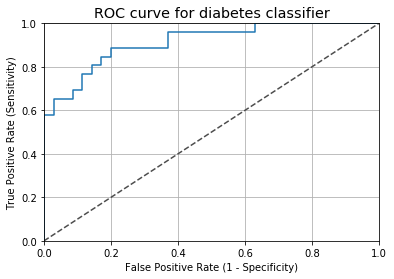auc(fpr, tpr)
#0.9131868131868132


## 结果解释

### 1.重要性贡献

Permutation importance是理解机器学习模型的第一个工具，它对验证数据集中的各个变量进行打乱(在一个模型被拟合之后)，并观察其对准确性的影响。

perm = PermutationImportance(model, random_state=1).fit(X_test, y_test)
eli5.show_weights(perm, feature_names = X_test.columns.tolist())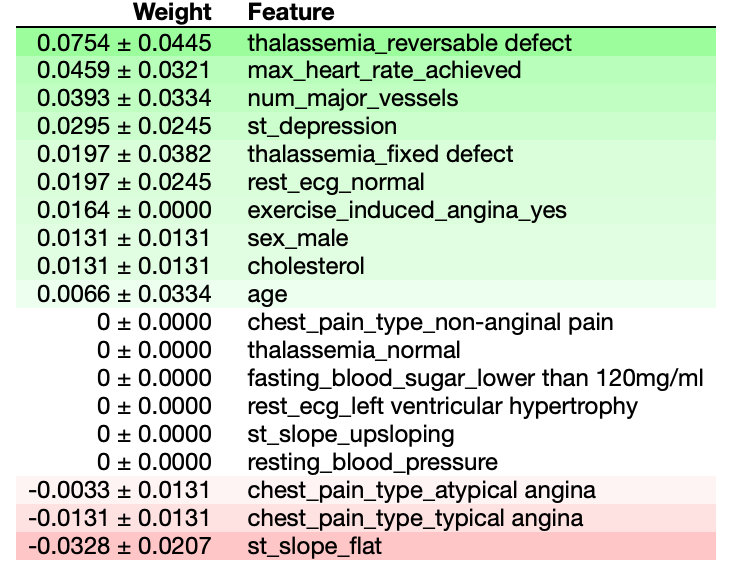import eli5 #for purmutation importance
from eli5.sklearn import PermutationImportance


### 2.单个变量变化对结果的影响

Partial Dependence Plot查看部分变量的改变对结果的影响。这些图在一个值范围内改变一行中的单个变量，并查看它对结果的影响。它对几行这样做，并绘制平均效果。让我们来看看’num_major_vessel '变量，它位于排列重要性列表的顶部。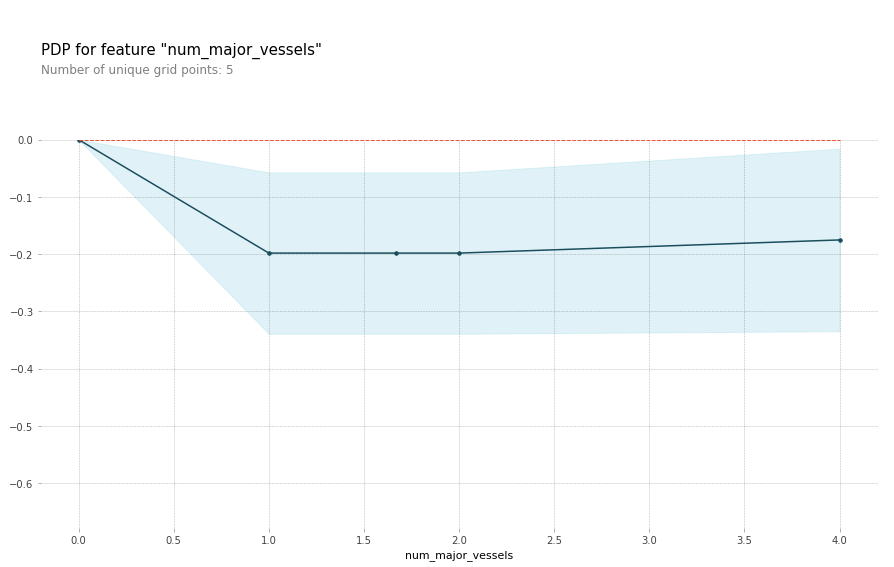from pdpbox import pdp, info_plots #for partial plots
pdp_dist = pdp.pdp_isolate(model=model, dataset=X_test, model_features=base_features, feature=feat_name)

pdp.pdp_plot(pdp_dist, feat_name)
plt.show()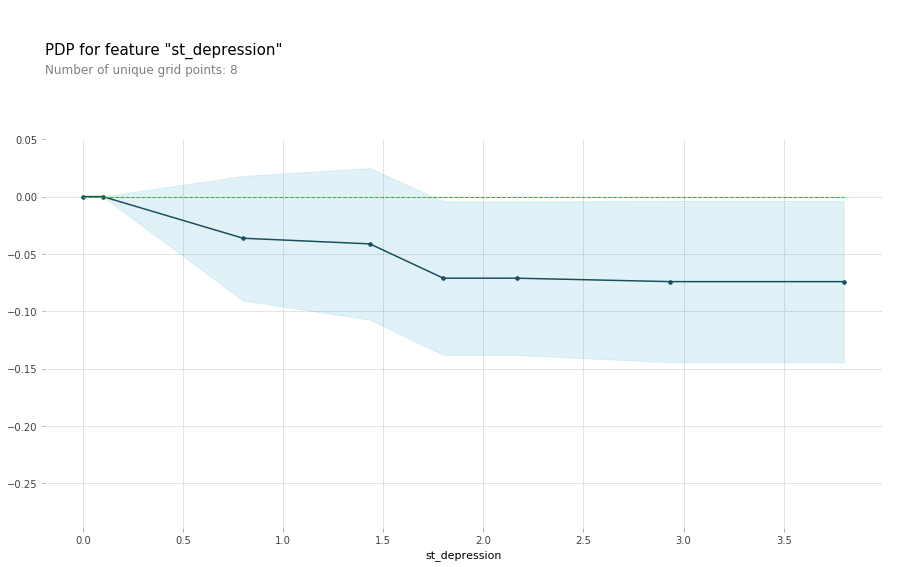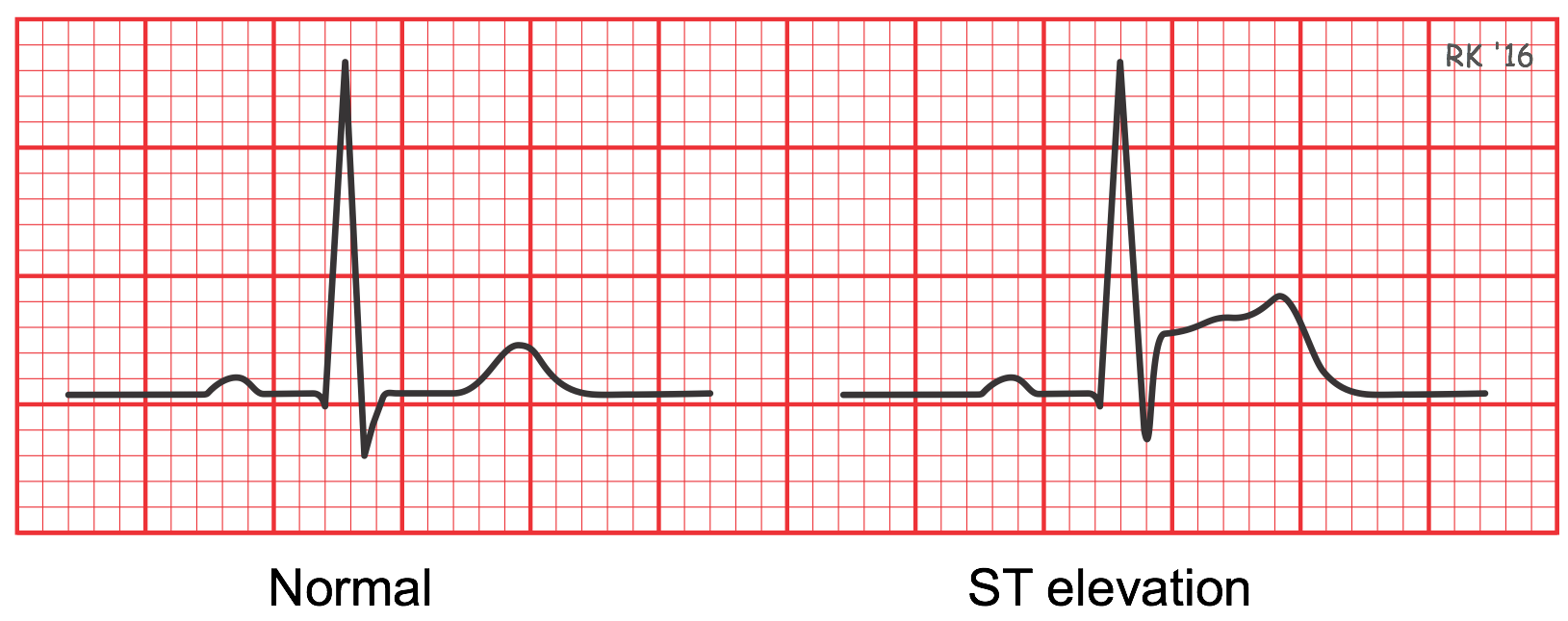inter1  =  pdp.pdp_interact(model=model, dataset=X_test, model_features=base_features, features=['st_slope_upsloping', 'st_depression'])

pdp.pdp_interact_plot(pdp_interact_out=inter1, feature_names=['st_slope_upsloping', 'st_depression'], plot_type='contour')
plt.show()

inter1  =  pdp.pdp_interact(model=model, dataset=X_test, model_features=base_features, features=['st_slope_flat', 'st_depression'])

pdp.pdp_interact_plot(pdp_interact_out=inter1, feature_names=['st_slope_flat', 'st_depression'], plot_type='contour')
plt.show()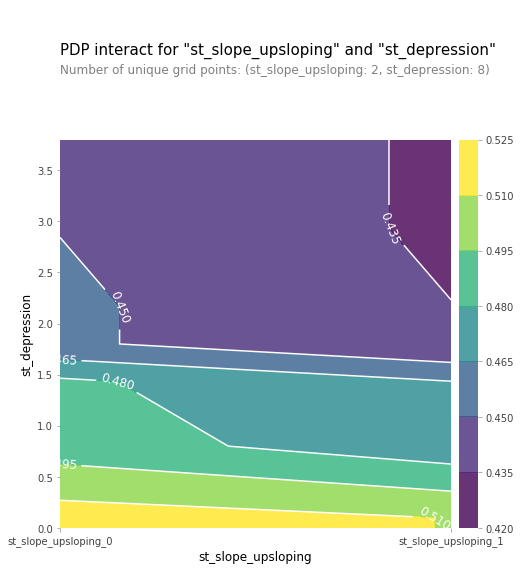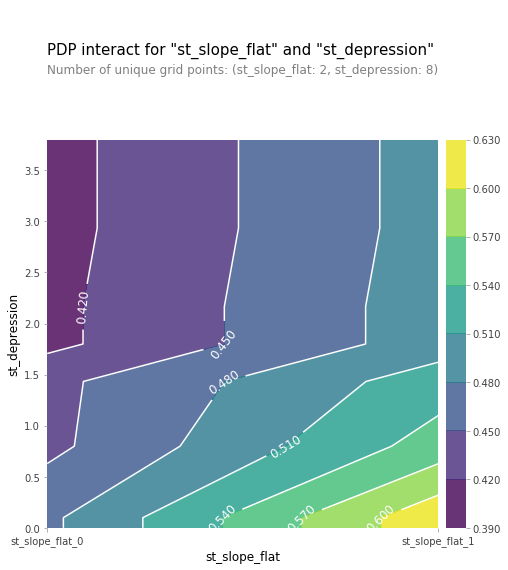### 3.使用SHAP值查看每一行数据变量值的影响

explainer = shap.TreeExplainer(model)
shap_values = explainer.shap_values(X_test)

shap.summary_plot(shap_values, X_test, plot_type="bar")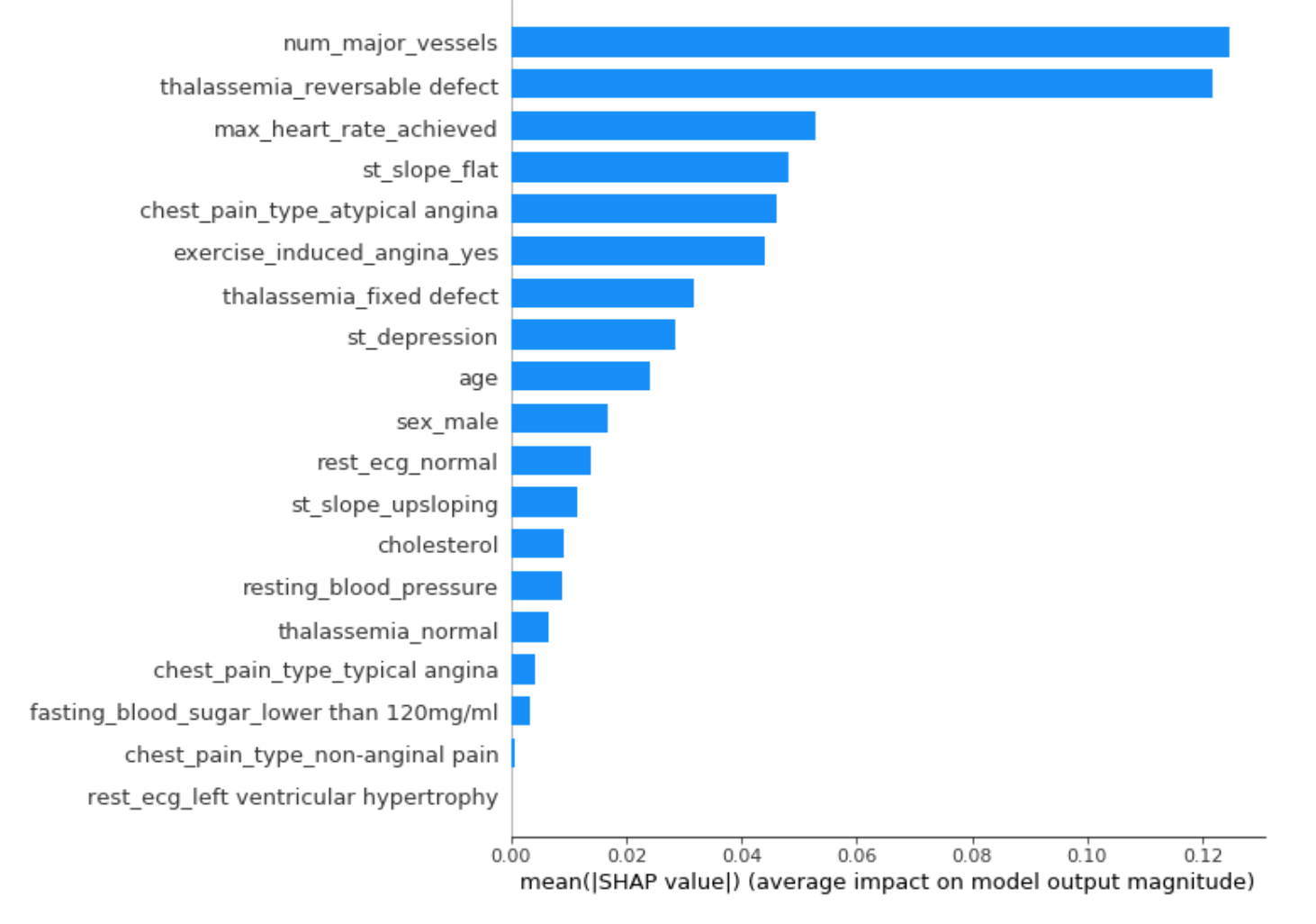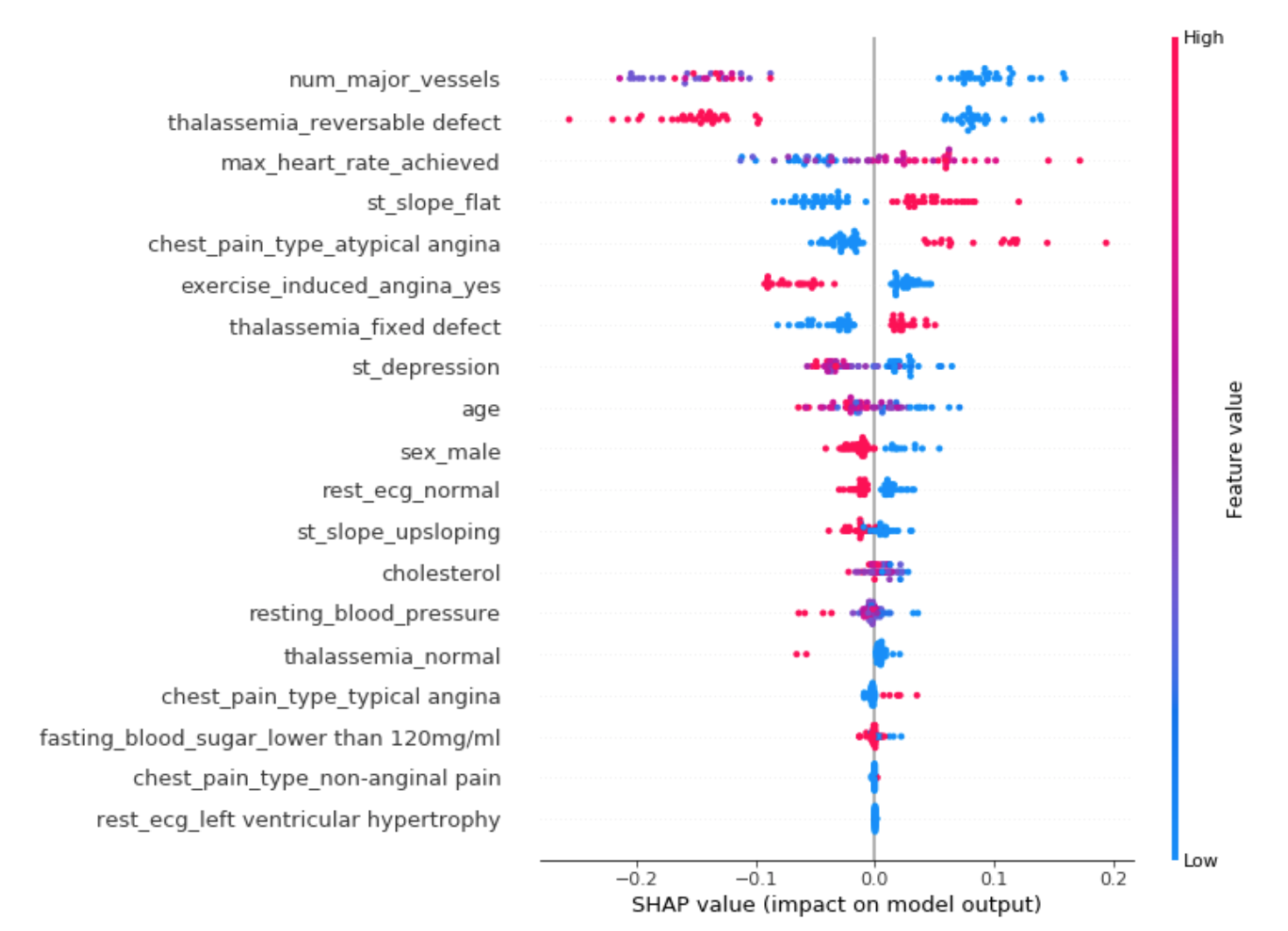def heart_disease_risk_factors(model, patient):
explainer = shap.TreeExplainer(model)
shap_values = explainer.shap_values(patient)
shap.initjs()
return shap.force_plot(explainer.expected_value, shap_values, patient)

data_for_prediction = X_test.iloc[1,:].astype(float)
heart_disease_risk_factors(model, data_for_prediction)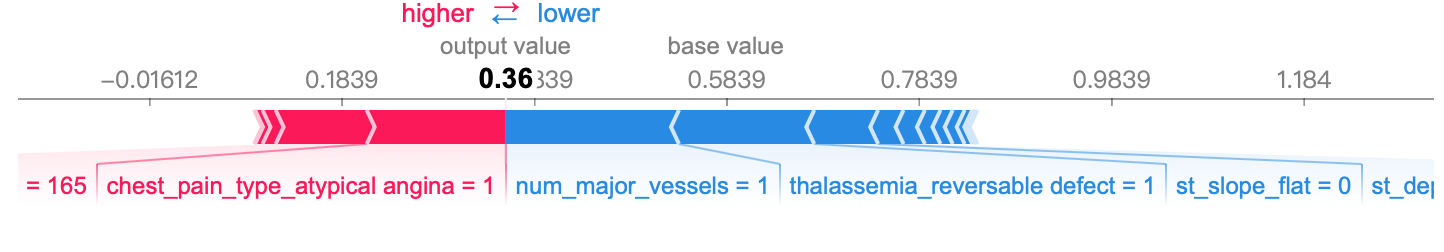data_for_prediction = X_test.iloc[3,:].astype(float)
heart_disease_risk_factors(model, data_for_prediction)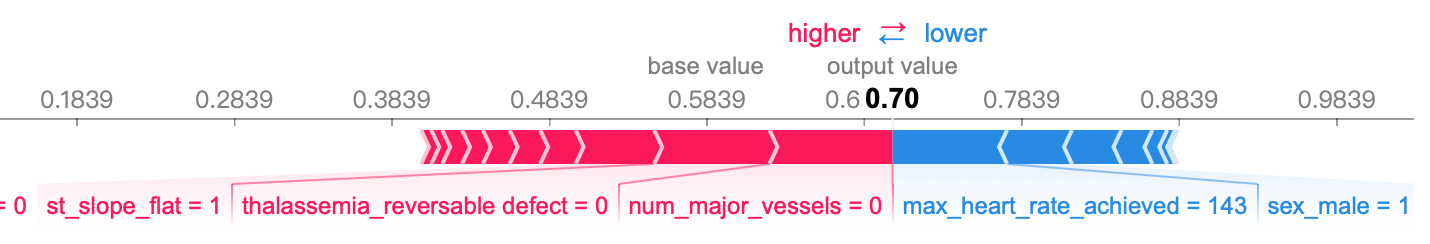ax2 = fig.add_subplot(224)
shap.dependence_plot('num_major_vessels', shap_values, X_test, interaction_index="st_depression")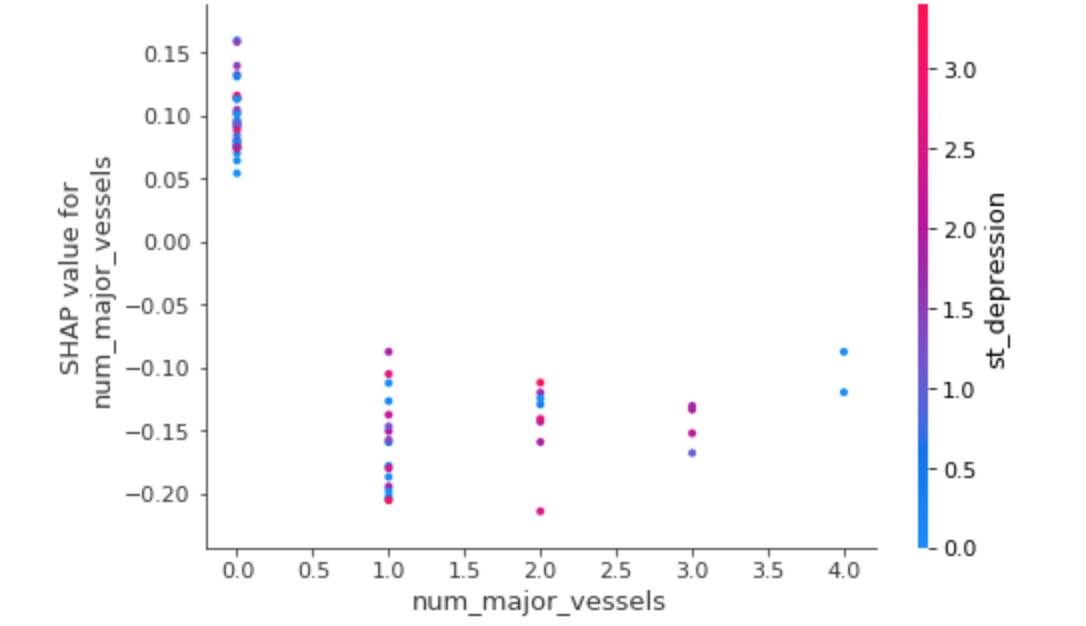shap_values = explainer.shap_values(X_train.iloc[:50])
shap.force_plot(explainer.expected_value, shap_values, X_test.iloc[:50])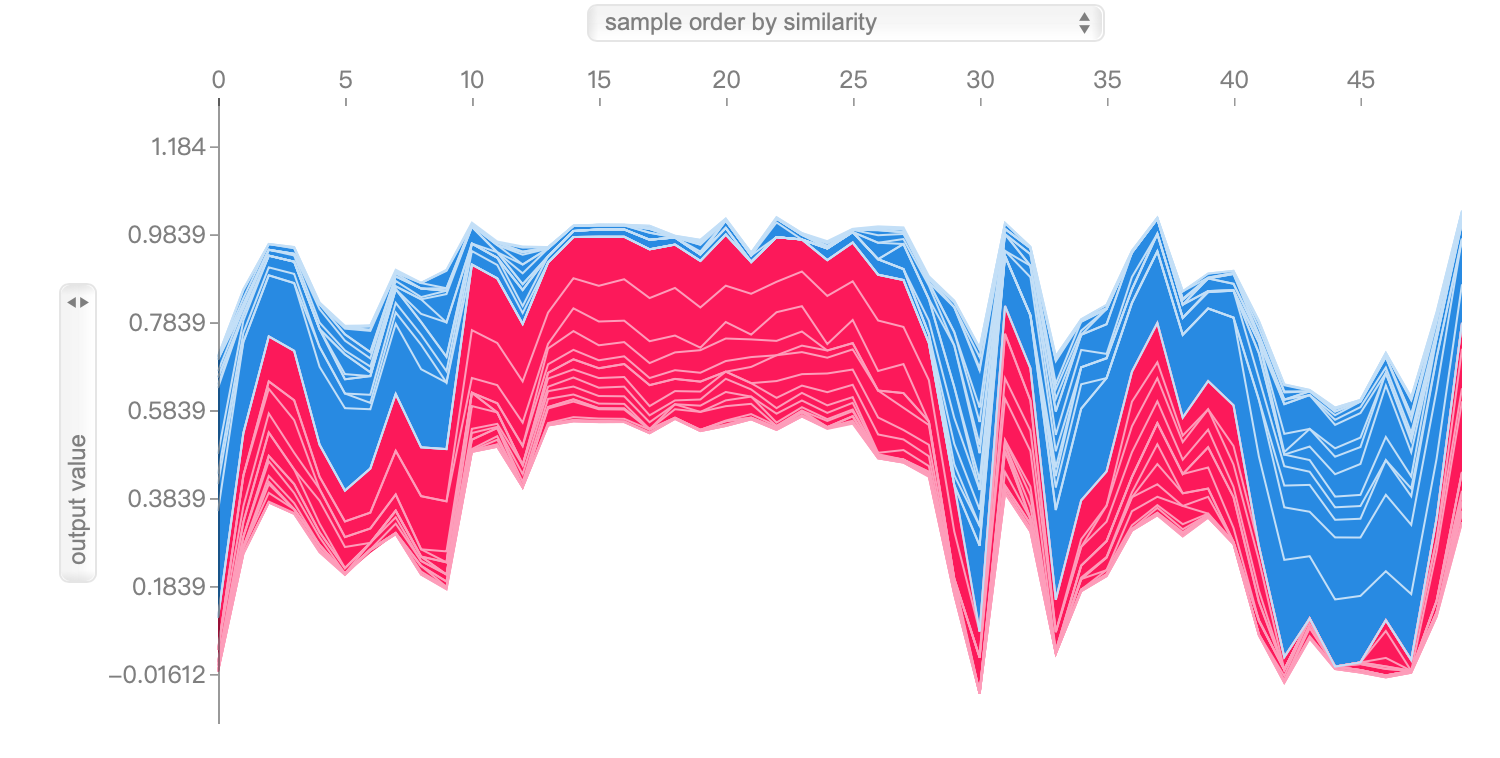## 小结

### 2，在实现过程中有哪些不常见，或者重要的细节？以及在对结果的解释有什么亮点？

Partial Dependence Plot查看部分变量的改变对结果的影响03-223820
02-192709
04-137309
11-102万+
05-06684
03-06
02-215900
12-191838
06-141万+
04-20945
04-071447
01-051万+
03-312902
05-251582
02-15